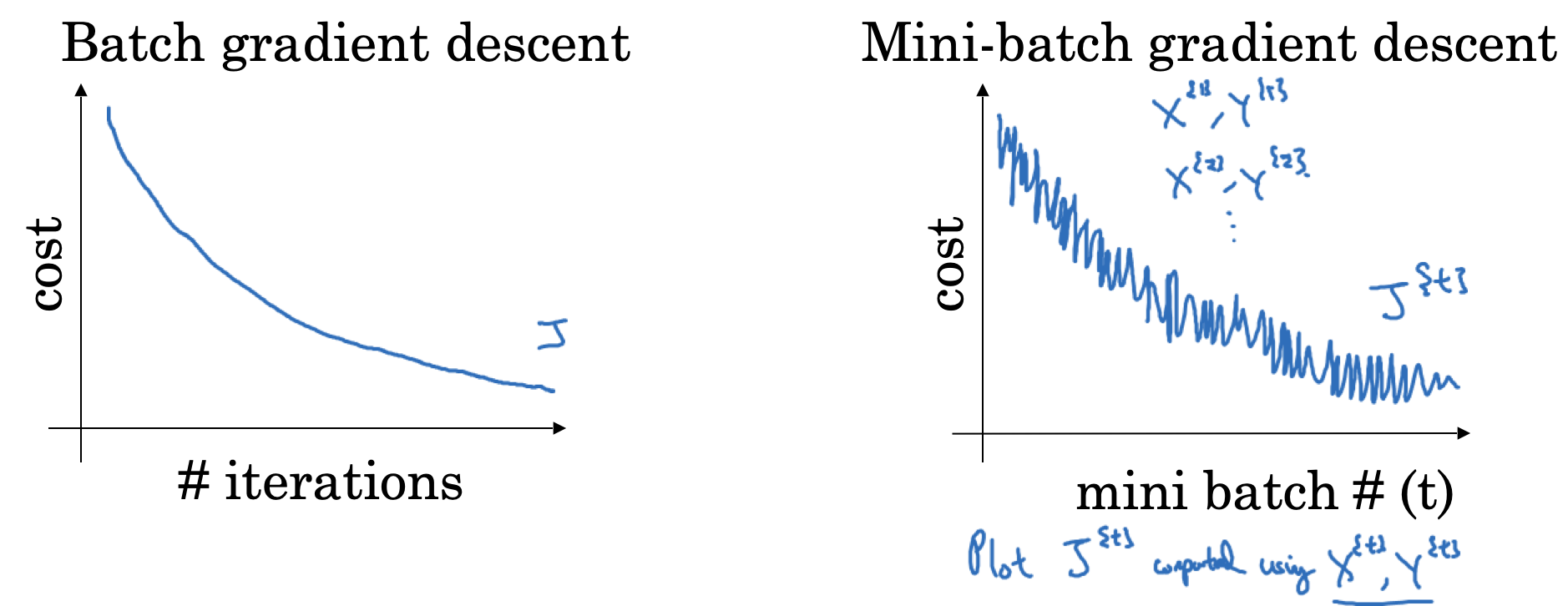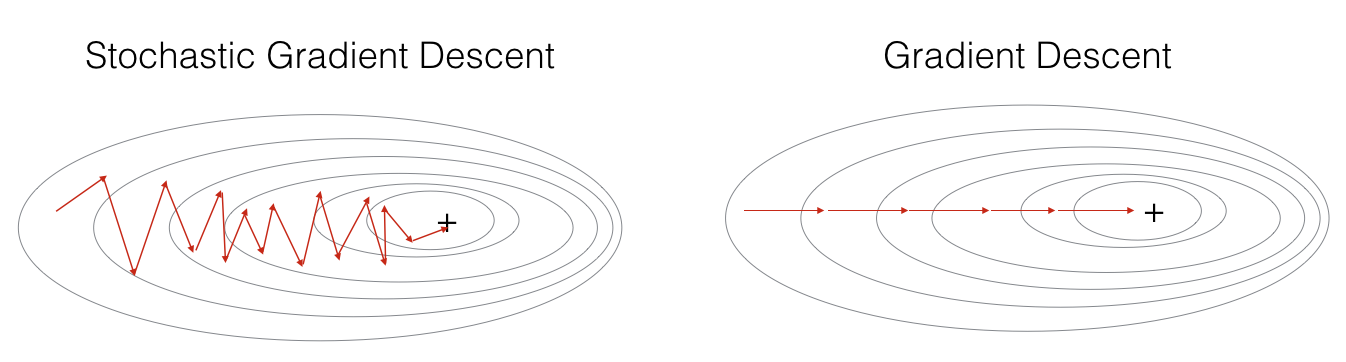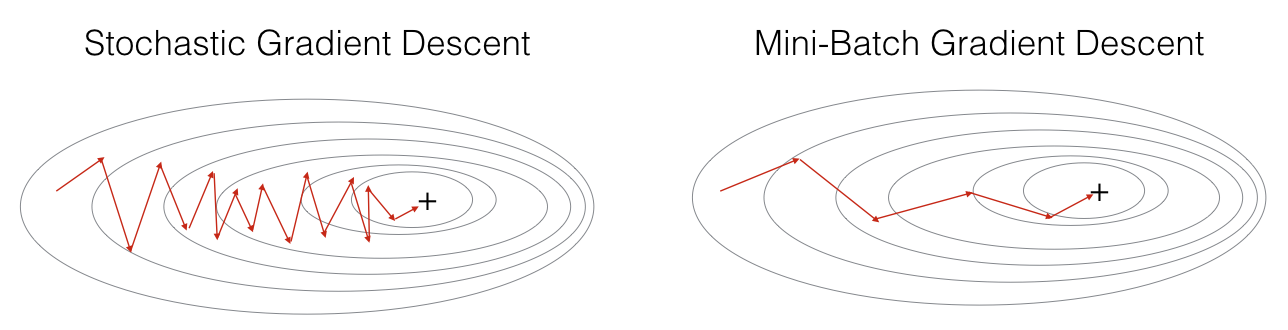# Optimization Algorithms• 当batch size为m的时候，称为Batch Gradient Descent，此时$(X^1, Y^1) = (X, Y)$
• 当batch size为1的时候，称为Stochastic Gradient Descent，此时$(X^1, Y^1) = (x^{(1)}, y^{(1)}), …, (X^{{t}}, Y^{{t}}) = (x^{(t)}, y^{(t)})$1. 如果训练样本很小(m<2000)，使用Batch Gradient Descent
2. 如果使用Mini Batch, m可选取2的阶乘，比如64, 128, 256, 512, 1024
3. 注意单个batch所产生的运算量(forward和backward)是否能被加载到当前的内存中

$\beta$在0和1之间，当$\beta$越大，曲线平滑，平均的样本数越多，反之曲线波动大，平均样本越少，如下图所示，分别是$\beta$为0.98，0.9和0.5时的曲线